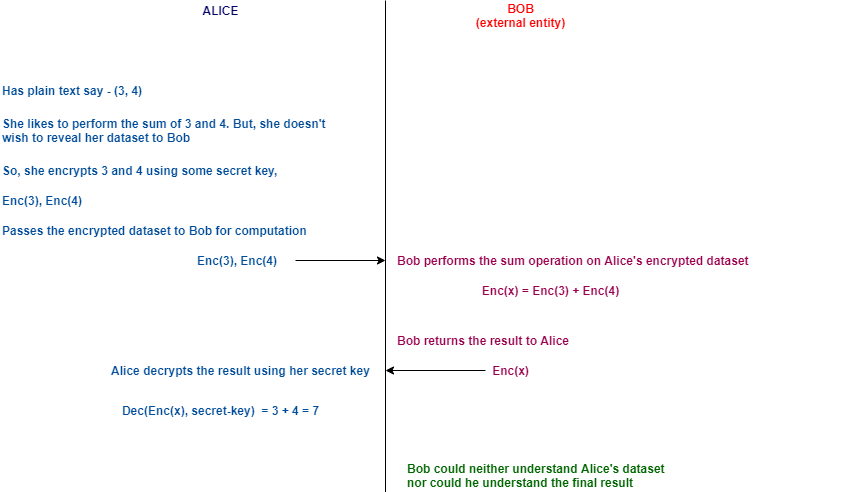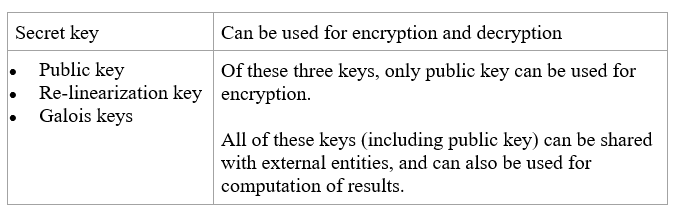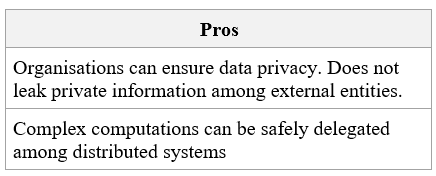Search

# Homomorphic Encryption

What is Homomorphic Encryption?

Homomorphic encryption is a technology that allows complex mathematical computations to be performed on the encrypted data, without ever decrypting it.

Using Homomorphic encryption, we can have other entities perform complex operations on our behalf, without giving them access to our personal/private/raw data, and get back the encrypted result.

The encrypted result thus obtained, when decrypted would be same as you would get if the operation was performed on the plain text. Decryption of results requires a secret key and as such, external entities (service providers) will not be able to comprehend the result that they would return to us.Figure 1: Basic understanding of homomorphic encryption

1. Addition and multiplication operations are supported since 2009

2. 109x speedup since 2009

3. Still 103 - 106 times slower than unencrypted operations

# Some real time examples

1. Locating nearby restaurants:

Most of us use Google maps for this purpose. In order to get the result, we end up sharing a lot of our private information such as - our location coordinates, time of the query, network details, etc. If we were to use Homomorphic encryption for this scenario, we would provide an encrypted query to Google. Google in turn would perform the required operations on the encrypted query and return the encrypted result to us, without knowing which information it returned to us, thereby, ensuring the privacy of our data at all stages of communication.

2. Healthcare Service Providers:

Nowadays, various kinds of health services are offered by various service providers in India as well as other countries. While subscribing for such a service, we end up giving away vital information such as our DNA. Using our DNA, one may be able find out about our genes, inheritance, etc.

This can be avoided very easily with the help of Homomorphic encryption wherein, the service providers will receive only encrypted information from their subscribers.

3. Internet of Things (IoT):

IoT consists of different devices. Generally, due to their low computing capabilities, they are connected to several gateways, and cloud with several servers to manage their data. Gateway receives messages from the sensors, encrypts them using homomorphic encryption and forwards them to the cloud. The cloud servers stores these cipher texts and performs required computations on them.

# Schemes

Some of the popular Homomorphic encryption schemes are as follows,

1. TFHE (Fast Fully Homomorphic Encryption over the Torus):

It is an open source software distributed under the terms of the Apache 2.0 license. This library implements logic gates on bits, and Fast Fourier Transformation. In simple words, their computations are modelled around Boolean circuits.

For example, consider a function F that takes two inputs - x and y and computes x + x*y. This can be represented as shown in the figure below.Figure 2: Basic THFE Circuit to compute x + (x * y)

In the above diagram, x and y are the inputs (private data) supplied by the client to the server that knows how to evaluate some function F (x, y). The client doesn't want the server to understand what x and y are.

It can evaluate circuits having both addition and multiplication gates. Also, it can have unlimited circuit depths, making it suitable for deep learning applications.

Although many variants of this scheme were proposed during the last decade, it has been very difficult to implement this in practice. However, Craig Gentry has shown in his recent study, how one may be able to implement this using what is called as the bootstrapping technique.

1. BGV (Brakerski-Gentry-Vaikuntanathan):

This scheme shares second place for efficiency, alongside CKKS. It is recognized as the most efficient scheme when performing the same set of operations on multiple cipher texts at once. It is known to be additively and multiplicatively homomorphic. Their computations are modeled on modular arithmetic or clock arithmetic. They are well suited for computations involving integer arithmetic and scalar multiplication.

2. CKKS (Cheon-Kim-Kim-Song):

It is similar to BGV. Unlike BGV, they approximate arithmetic on the vectors. It models its computations as floating-point arithmetic. There are two types of floating-point representations. They are,

a. Fixed point representation:

Use fixed number of bits for integral part and fractional part.

b. IEEE Floating point number representation:

It does not reserve a specific number of bits for fractional part or the integral part. Instead it reserves certain number of bits for the number and certain number of bits to indicate where within that number the decimal place has to be placed.

Of the two representations, CKKS prefers Fixed point representation over IEEE Floating Point representation as the latter is considered slightly more complicated.

CKKS scheme commonly used in places where precision has less importance. For example - polynomial approximation, machine learning models, etc.

Summary tableAll of the above-mentioned schemes are classified as second-generation fully homomorphic encryption schemes.

# Stages

Use of Homomorphic encryption involves five stages. They are as follows,

1. Setup

a. Choosing an appropriate scheme

b. Deciding on the security parameters

c. Choosing functional parameters

2. Key Generation3. Encryption4. Evaluation

Required operations on the cipher text. The output from this step will also be a cipher text.

5. Decryption

Decryption of cipher text using a secret key, to get back the intended result.

# Working principle

The basic working principle of Homomorphic Encryption is depicted in the following block diagrams,Figure 3: Homomorphic Encryption - working principle

Whether it’s a single-digit number or a vector of numbers, the ciphertext is always a random degree polynomial. It is not possible for one to determine the plain text, given the ciphertext.

Computation on the cipher texts are performed as follows,

Encryption (1, 2, 3, 4) + Encryption (1, 2, 3, 4) → Encryption (2, 4, 6, 8)

Encryption (1, 2, 3, 4) * Encryption (1, 2, 3, 4) → Encryption (2, 4, 9, 16)

# Pros and Cons of Homomorphic EncryptionReferences

· IBM

What Is Homomorphic Encryption And How Can You Use It In Practice

· Microsoft Research

Intro to Homomorphic Encryption

· Wikipedia

Homomorphic Encryption

· Medium.com

Homomorphic Encryption for Beginners

· Baffle.io

· Ayoub Benaissa's Blog

Homomorphic Encryption Types

· nucypher.com

Fully Homomorphic Encryption for Engineers

· kontron.com

Homomorphic Encryption Use Case